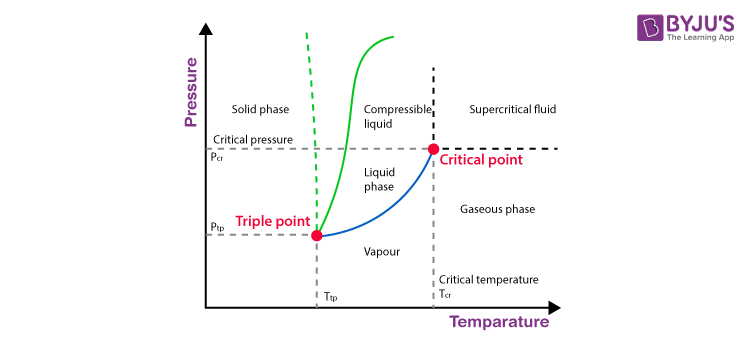# Equilibrium Involving Dissolution Of Solid Or Gas In Liquid

## What is Equilibrium?

Everything in the universe tries to attain stability by lowering its energy. This can be achieved by various physical and chemical processes. All reactions proceed unit they reach certain minimum energy in accordance with their surroundings. They reach the state where there is no visible change in the system and remain that way unless disturbed. This state is called equilibrium. In this state, the rate of the forward reaction is equal to the rate of the backward reaction.

### Equilibrium involving Dissolution of Solid or Gases in Liquids:

#### Solids in liquids:

Have you ever tried dissolving sugar in water? Can you dissolve any amount of sugar? After a certain quantity, the added sugar gets deposited. We call a solution saturated when the solution cannot dissolve any more solute in it, at a given temperature. In a saturated solution, a dynamic equilibrium exists between solute molecules in the solid-state and in the solution at a given temperature. The increase in temperature increases the solubility of the solution i.e. solubility depends on temperature.

$$\begin{array}{l}~~~~~~~~~~~~~~~\end{array}$$
Sugar (solution)  ⇌ sugar (solid)
$$\begin{array}{l}~~~~~~~~~~~~~~~\end{array}$$
Rate of dissolution of sugar = Rate of crystallisation of sugar

#### Gases in Liquids:

Why do you think the carbonated drinks (cold drinks) are refrigerated? What will happen when the carbonated cold drink is at room temperature? Do you observe any difference? Let’s try to understand the reason behind this.

We notice that the gas fizzing out from the cold drink at room temperature is more than the drink that is refrigerated. This happens because of the solubility of carbon dioxide at different pressures. The relation between the quantity of gas dissolved can be given by Henry’s Law. This law states that the mass of a gas dissolved in the given amount of solvent at any temperature is proportional to the pressure of the gas above the solvent. The solubility of gases decreases with an increase in temperature.

$$\begin{array}{l}~~~~~~~~~~~~~~~\end{array}$$
(Mass of the gas) α (pressure of the gas)
$$\begin{array}{l}~~~~~~~~~~~~~~~\end{array}$$
m α p
$$\begin{array}{l}~~~~~~~~~~~~~~~\end{array}$$
mm = kHpWhere kH is the proportionality constant, called Henry’s law constant and depends on the temperature. The cold drink is sealed under a pressure of the gas (generally higher than atmospheric pressure) where its is high in the solvent (water). When the drink is opened, it is exposed to the atmosphere (lower pressure than the drink) due to which some CO2 gas escapes the bottle to establish the new dynamic equilibrium in accordance with low pressure, namely its partial pressure in the atmosphere. If the drink is exposed to the surrounding most of the dissolved gas escapes because the solubility is low under low pressure and high temperature (when compared to the refrigerator). Hence, more gas pops out of a drink kept at room temperature than a drink which is just taken out from a refrigerator.

## Frequently Asked Questions – FAQs

Q1

### What is equilibrium and what is an example?

The state in which the measurable properties of the system such as pressure, density, color or concentration do not undergo any further noticeable changes with time under a given set of conditions is said to be a state of equilibrium. Example : 2HI ⇋ H2 + I2
Q2

### What is KP and KC in equilibrium?

Kp is equilibrium constant used when partial pressures of the species are given and Kc is equilibrium constant used when equilibrium concentrations are expressed in molarity.
Q3

### Why is equilibrium important in chemistry?

When the quantities of reactants and products are stable – their ratio does not change a chemical reaction is in equilibrium. This suggests that the reaction has reached a point where the reactant and product amounts remain constant over time, since the forward and backward reactions are at the same rate.
Q4

### What is pH formula?

pH is described as the negative of the logarithm of the molar hydronium-ion concentration. pH formula is -log [H3O+].

To learn more about equilibrium constant and the law of mass action subscribe to BYJU’S – The Learning App

Take up a quiz on Equilibrium Involving Dissolution Of Solid Or Gas In Liquid